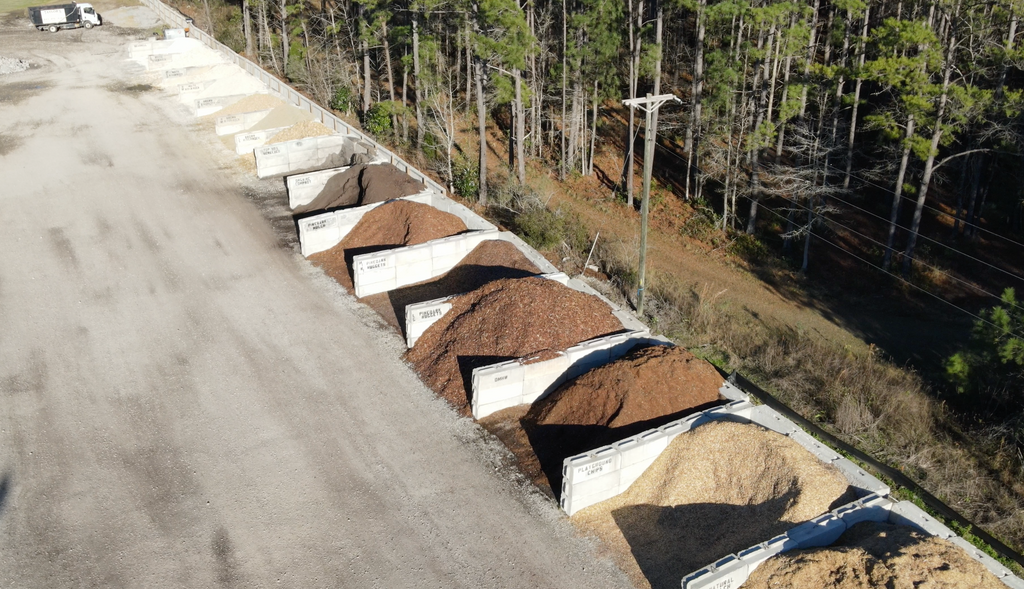# How to Calculate How Much Mulch You Need for Your Landscaping ProjectReady to transform your yard into a beautiful outdoor oasis? The first step is to calculate how much mulch you'll need to give your plants the perfect finishing touch. Don't let math scare you - we'll walk you through the process and make it as easy as can be.

## Learn the Easy Way to Calculate How Much Mulch You Need

Calculating the amount of mulch you need may seem intimidating at first, but it's actually quite simple. All you need is a measuring tape and a calculator.

First, measure the length and width of the area you want to cover with mulch. Be sure to measure in feet, as most mulch is sold by the cubic yard.

Next, decide on the depth of the mulch layer you want to apply. This is usually measured in inches. A general rule of thumb is to use a 2-3 inch layer for a decorative finish, and a 4-6 inch layer for weed control.

Now, it's time to do some math! Use the following formula to calculate the volume of mulch you need:

Mulch volume = length x width x (depth / 12 inches/ft) = cubic feet

This formula may look complicated, but it's really just a way of converting the depth of the mulch layer (measured in inches) to feet, so it can be used in the calculation.

To convert the volume to cubic yards, divide the volume in cubic feet by 27 (the number of cubic feet in a cubic yard). This will give you the total number of cubic yards of mulch you need.

## Real World Example of Calculating the Right Amount of Mulch to Buy

For example, let's say you want to cover an area that is 20 feet long and 10 feet wide, and you want to apply a mulch layer that is 3 inches deep. Using the formula above, you would calculate the volume of mulch you need as follows:

Mulch volume = 20 ft x 10 ft x (3 inches / 12 inches/ft) = 50 cubic feet

To determine how many cubic yards of mulch you need, divide the volume in cubic feet by 27:

Mulch needed = 50 cubic feet / 27 cubic feet/cubic yard = 1.85 cubic yards

You'll need to round up to the nearest whole number, so you'll need to buy 2 cubic yards of mulch.

Now that you know how to calculate the amount of mulch you need, it's time to get shopping! Head on over to Taylor's Landscape Supply and try out our Mulch Calculator to make your purchase a breeze.

### Try Taylor's Mulch Calculator

To calculate how much mulch you need, please provide the following information:

• Length of the area you want to cover with mulch (measured in feet)
• Width of the area you want to cover with mulch (measured in feet)
• Depth of the mulch layer you want to apply (measured in inches)"

### 1 comment

• Danny Allen

Live in the Southside of Savannah – need to cover 2700 sq ft with pine bark – how much delivered